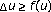# Second-order estimates for boundary blowup solutions of special elliptic equations

## Abstract

We find a second-order approximation of the boundary blowup solution of the equation, with, in a bounded smooth domain. Furthermore, we consider the equation. In both cases, we underline the effect of the geometry of the domain in the asymptotic expansion of the solutions near the boundary.

## References

1. Andersson L, Chruściel PT: Solutions of the constraint equations in general relativity satisfying "hyperboloidal boundary conditions". Dissertationes Mathematicae (Rozprawy Matematyczne) 1996, 355: 1-100.

2. Anedda C, Buttu A, Porru G: Boundary estimates for blow-up solutions of elliptic equations with exponential growth. to appear in Proceedings Differential and Difference Equations

3. Anedda C, Porru G: Higher order boundary estimates for blow-up solutions of elliptic equations. to appear in Differential Integral Equations

4. Bandle C: Asymptotic behaviour of large solutions of quasilinear elliptic problems. Zeitschrift für Angewandte Mathematik und Physik 2003,54(5):731-738. 10.1007/s00033-003-3207-0

5. Bandle C, Giarrusso E: Boundary blow up for semilinear elliptic equations with nonlinear gradient terms. Advances in Differential Equations 1996,1(1):133-150.

6. Bandle C, Marcus M: "Large" solutions of semilinear elliptic equations: existence, uniqueness and asymptotic behaviour. Journal d'Analyse Mathématique 1992, 58: 9-24.

7. Bandle C, Marcus M: On second-order effects in the boundary behaviour of large solutions of semilinear elliptic problems. Differential and Integral Equations 1998,11(1):23-34.

8. Bandle C, Marcus M: Dependence of blowup rate of large solutions of semilinear elliptic equations, on the curvature of the boundary. Complex Variables. Theory and Application 2004,49(7–9):555-570.

9. Berhanu S, Porru G: Qualitative and quantitative estimates for large solutions to semilinear equations. Communications in Applied Analysis 2000,4(1):121-131.

10. Bieberbach L:und die automorphen Funktionen. Mathematische Annalen 1916,77(2):173-212. 10.1007/BF01456901

11. del Pino M, Letelier R: The influence of domain geometry in boundary blow-up elliptic problems. Nonlinear Analysis. Theory, Methods & Applications. Series A: Theory and Methods 2002,48(6):897-904.

12. Gilbarg D, Trudinger NS: Elliptic Partial Differential Equations of Second Order, Grundlehren der mathematischen Wissenschaften. Volume 224. Springer, Berlin; 1977:x+401.

13. Greco A, Porru G: Asymptotic estimates and convexity of large solutions to semilinear elliptic equations. Differential and Integral Equations 1997,10(2):219-229.

14. Keller JB:On solutions of. Communications on Pure and Applied Mathematics 1957, 10: 503-510. 10.1002/cpa.3160100402

15. Lazer AC, McKenna PJ: Asymptotic behavior of solutions of boundary blowup problems. Differential and Integral Equations 1994,7(3-4):1001-1019.

16. Osserman R:On the inequality. Pacific Journal of Mathematics 1957,7(4):1641-1647.

## Author information

Authors

### Corresponding author

Correspondence to Giovanni Porru.

## Rights and permissions

Reprints and Permissions

Anedda, C., Buttu, A. & Porru, G. Second-order estimates for boundary blowup solutions of special elliptic equations. Bound Value Probl 2006, 45859 (2006). https://doi.org/10.1155/BVP/2006/45859